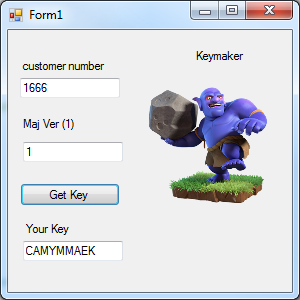## Sunday, June 23, 2019

### How to Make A Keymaker in C-Sharp

You can reverse engineer .Net programs to find out how other software developers protect their applications.  It is a good exercise that will teach you how to make a better software protection scheme.  The first thing to do is get a good set of reverse engineering programs. I recommend dotPeek32. DotPeek32 can open up a .Net program and show you the source code.  Dot.net programs are merely interpreted and not true machine code. They are Microsoft's famous JustInTime compiled with CLR run time code. Using DotPeek or RedGate .Net Reflector, you can easily reverse engineer any application.  My simple keymaker serves as an example of how you can make keymakers using one that I found inside an obscure industrial service application.  You could use the same things in your own application, although I would recommend extending it to 32 characters.The Simple keymaker UI
the code shown below is the called Sample_Keygen.Form1. It is the event handler for the "Get Key" button.  It works by taking a four digit customer number and 2 digit major version number as inputs, then applying an offset to the customer number strings and version number strings and spreading them over nine (9) characters of an char array.  The second and fourth characters are just random characters that are not used.   In actual operation, the output is stored in TextBox3 and is then given to the customer to use in his own application.  This same function is used in the protected application, and the second and fourth characters are not compared against the inputted key value.  It is a simple and effective way to protect an application against software piracy.

```using System;
using System.Collections.Generic;
using System.ComponentModel;
using System.Data;
using System.Drawing;
using System.Linq;
using System.Text;
using System.Windows.Forms;
using Microsoft.VisualBasic;
using Microsoft.VisualBasic.CompilerServices;

// using this dll
// C:\Program Files (x86)\Reference Assemblies\Microsoft\Framework\.NETFramework\v4.0
//
namespace Sample_Keygen
{
public partial class Form1 : Form
{
public Form1()
{
InitializeComponent();
}

private void button1_Click(object sender, EventArgs e)
{
int num1;
object obj1;
int num2;
int CustNum = 0;
int VersionAPP = 1;
string Num, Ver;
char[] chArray = new char;
Num = textBox1.Text.ToString();
Ver = textBox2.Text.ToString();
if (!Int32.TryParse(Num, out NumNaja))
{
NumNaja = 0;
}
if (!Int32.TryParse(Ver, out VersionAPP))
{
VersionAPP = 1;
}

VBMath.Randomize();
string str1 = Microsoft.VisualBasic.Strings.Format((object)(double)CustNum, "0000");
string str2 = Microsoft.VisualBasic.Strings.Format((object)(double)VersionAPP, "00");
chArray = Microsoft.VisualBasic.Strings.Chr(checked((int)Math.Round(unchecked(Conversions.ToDouble(Microsoft.VisualBasic.Strings.Mid(str1, 1, 1)) * 2.0 + 65.0))));
chArray = Microsoft.VisualBasic.Strings.Chr(checked((int)Math.Round(unchecked((double)VBMath.Rnd() * 26.0 + 65.0))));
chArray = Microsoft.VisualBasic.Strings.Chr(checked((int)Math.Round(unchecked(Conversions.ToDouble(Microsoft.VisualBasic.Strings.Mid(str1, 3, 1)) * 2.0 + 65.0))));
chArray = Microsoft.VisualBasic.Strings.Chr(checked((int)Math.Round(unchecked((double)VBMath.Rnd() * 26.0 + 65.0))));
chArray = Microsoft.VisualBasic.Strings.Chr(checked((int)Math.Round(unchecked(Conversions.ToDouble(Microsoft.VisualBasic.Strings.Mid(str1, 2, 1)) * 2.0 + 65.0))));
chArray = Microsoft.VisualBasic.Strings.Chr(checked((int)Math.Round(unchecked(Conversions.ToDouble(Microsoft.VisualBasic.Strings.Mid(str1, 4, 1)) * 2.0 + 65.0))));
chArray = Microsoft.VisualBasic.Strings.Chr(checked((int)Math.Round(unchecked(Conversions.ToDouble(Microsoft.VisualBasic.Strings.Mid(str2, 1, 1)) * 2.0 + 65.0))));
chArray = Microsoft.VisualBasic.Strings.Chr(checked((int)Math.Round(unchecked(Conversions.ToDouble(Microsoft.VisualBasic.Strings.Mid(str2, 2, 1)) * 4.0 + 65.0))));
chArray = Microsoft.VisualBasic.Strings.Chr(checked(unchecked(checked(Microsoft.VisualBasic.Strings.Asc(chArray) - 65 + Microsoft.VisualBasic.Strings.Asc(chArray) - 65 + Microsoft.VisualBasic.Strings.Asc(chArray) - 65 + Microsoft.VisualBasic.Strings.Asc(chArray) - 65 + Microsoft.VisualBasic.Strings.Asc(chArray) - 65 + Microsoft.VisualBasic.Strings.Asc(chArray) - 65) % 26) + 65));
obj1 = (object)(Conversions.ToString(chArray) + Conversions.ToString(chArray) + Conversions.ToString(chArray) + Conversions.ToString(chArray) + Conversions.ToString(chArray) + Conversions.ToString(chArray) + Conversions.ToString(chArray) + Conversions.ToString(chArray) + Conversions.ToString(chArray));

textBox3.Text = obj1.ToString();
}
}
}
```